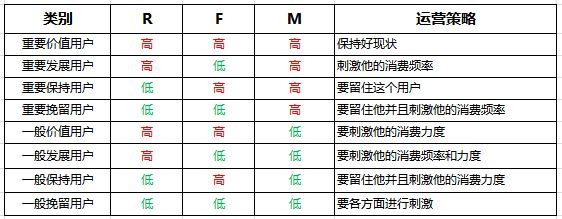# 方法篇｜如何成为一个运营大牛（一）：用户分层

• 用户画像：一般体现的是用户的客观属性。如性别、年龄、职业等，一般是不以用户的主观意愿所转移的。
• 用户分层：一般体现的是用户在产品上所处的状态。比如免费用户、活跃用户、付费用户、高额付费用户等，由于是“层”嘛，所以它有一个层级的概念，有一个状态递进的过程，大多是呈漏斗形的形状。而且用户的层级一般不会分的太多。
• 用户分群：一般体现的是用户的行为表现上。比如说频次低单价高、频次高单价低，这两种用户可能都属于高额付费用户，但表现形式不一样，所以所处的群也是不一样，所对应的运营策略也是不一样的。也可以认为用户分群是用户分层的进一步精细化过程。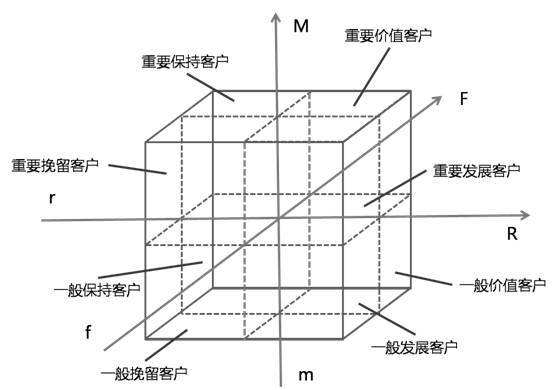RFM模型历史悠久，其理论知识这里就不阐述了，简单的说就是通过最近一次消费(Recency)、消费频率(Frequency)、消费金额(Monetary)这三个指标，然后把每个指标按照实际的情况，分成5档，一共形成了125类的用户。然后为了执行方便，把125类的用户归纳成8大类，如下图，最后根据这8大类用户的情况制定运营策略。（此处只选10条数据做实例）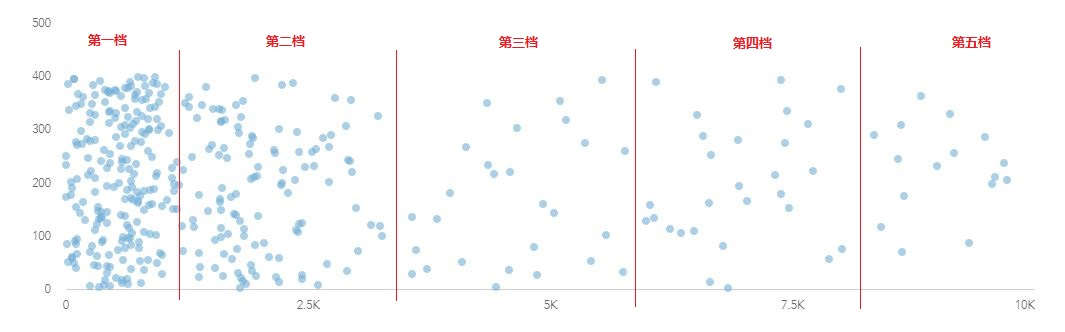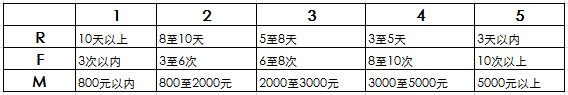• 如果B3>10,那么其R值为1，否则进入下一个判断；
• 如果B3>8,那么其R值为2，否则进入下一个判断；
• 如果B3>5,那么其R值为3，否则进入下一个判断；
• 如果B3>3,那么其R值为4，否则为5；

F3=IF(C3>10,5,IF(C3>8,4,IF(C3>6,3, IF(C3>3,2,1))))

G3= =IF(D3>5000,5,IF(D3>3000,4,IF(D3>2000,3, IF(D3>800,2,1))))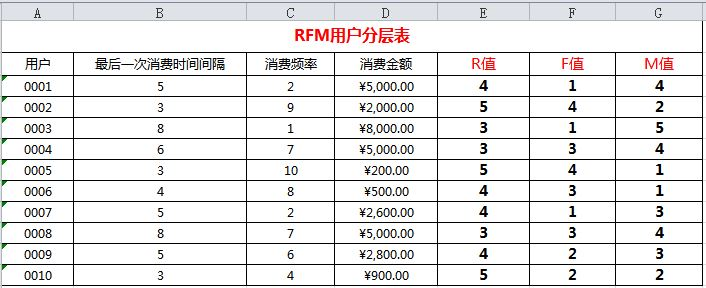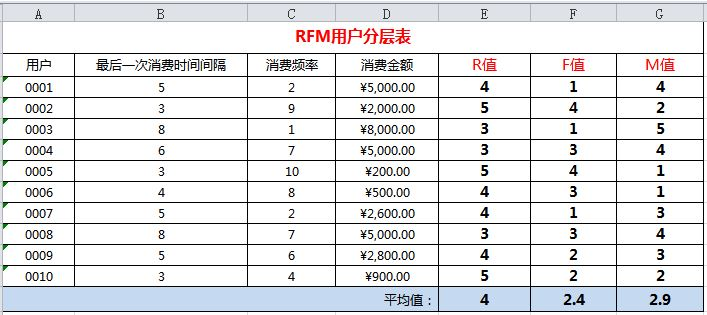• H3=IF(E3<E13,”低”,”高”)，同理，
• F高低值I3=IF(F3<F13,”低”,”高”)
• M高低值J3=IF(G3<G13,”低”,”高”)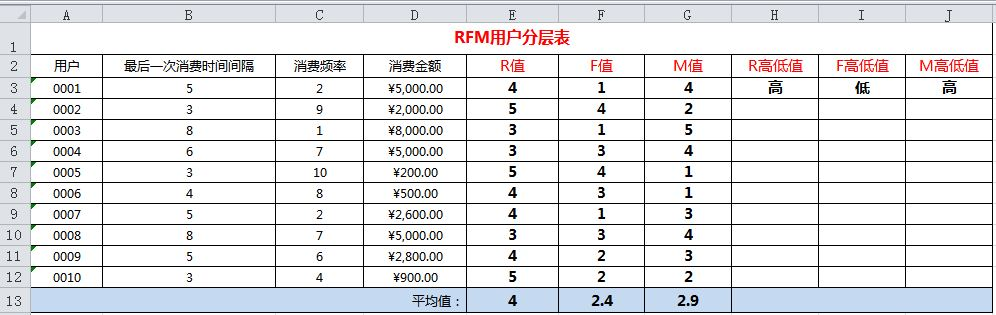• R高低值H3=IF(E3< \$E \$13,”低”,”高”)
• F高低值I3=IF(F3< \$F \$13,”低”,”高”)
• M高低值J3=IF(G3< \$G \$13,”低”,”高”)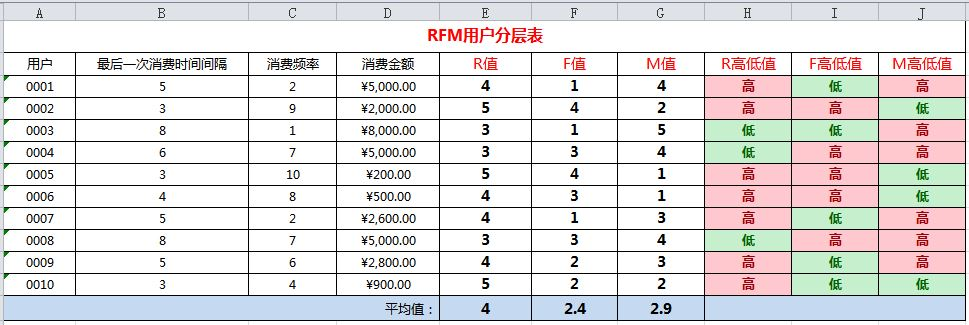接下来，我们就要根据我们的“R高低值”“F高低值”“M高低值”，自动计算出我们的用户层级拉。我们先加个表头“用户层级”。

• K3=IF(AND(H3=”高”,I3=”高”,J3=”高”),”重要价值用户”, IF(AND(H3=”高”,I3=”低”,J3=”高”),”重要发展用户”,
• IF(AND(H3=”低”,I3=”高”,J3=”高”),”重要保持用户”, IF(AND(H3=”低”,I3=”低”,J3=”高”),”重要挽留用户”,
• IF(AND(H3=”高”,I3=”高”,J3=”低”),”一般价值用户”, IF(AND(H3=”高”,I3=”低”,J3=”低”),”一般发展用户”,
• IF(AND(H3=”低”,I3=”高”,J3=”低”),”一般保持用户”,”一般挽留用户”)))))))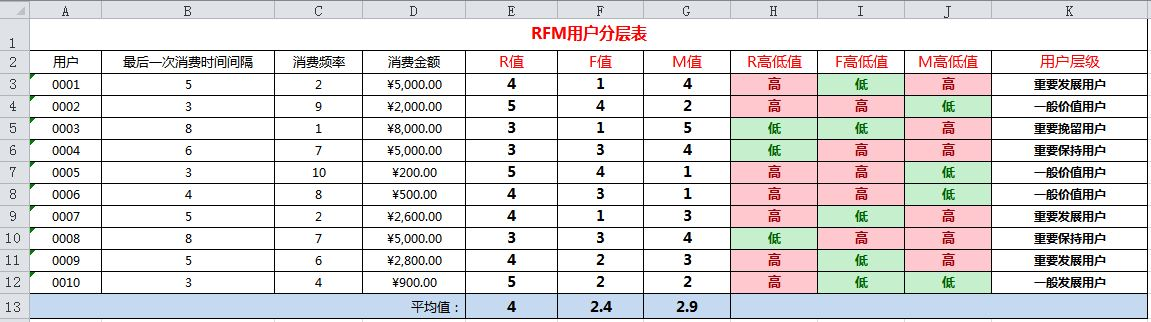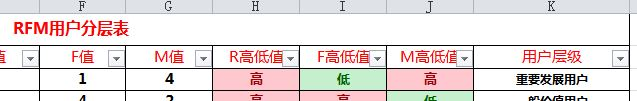## 第七步：形成图表

完成后上面六步之后，我们已经得到了完成用户分层之后的所有用户记录，这时我们需要做成图表的形式，开个会、做个汇报啥的，如下图：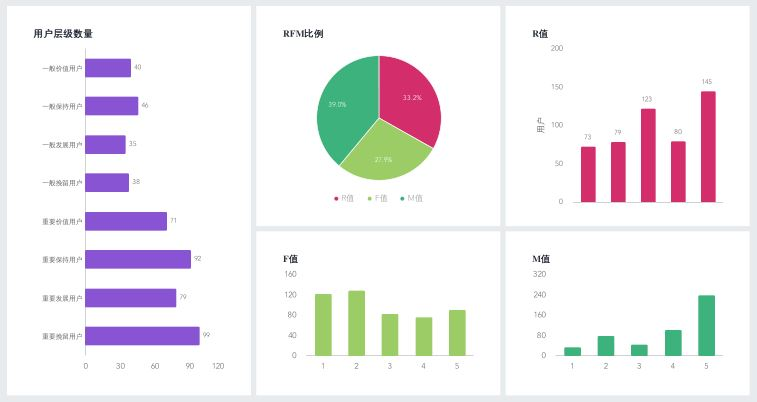## 第八步：制定运营策略